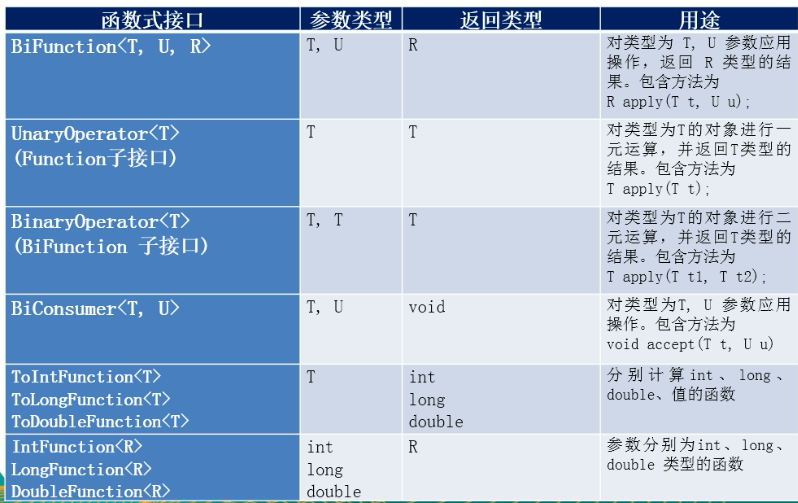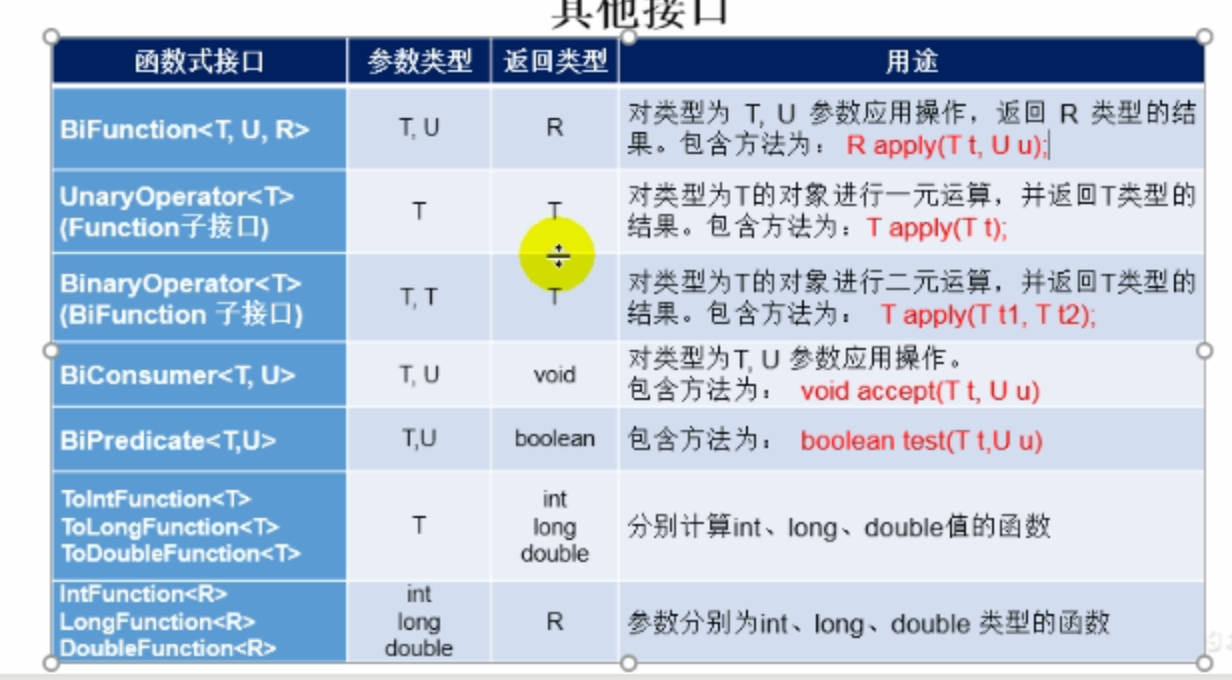• Consumer 消费型接口 消费对象 void accept(T t); Supplier 供给型接口 生成对象 T get(); Function<R,T> 函数型接口 指定特定功能 R apply(T t); Predicate 断言型接口 进行条件判断 boolean test(T t);

Consumer 消费型接口 消费对象
void accept(T t);
Supplier 供给型接口 生成对象
T get();
Function<R,T> 函数型接口 指定特定功能
R apply(T t);
Predicate 断言型接口 进行条件判断
boolean test(T t);

展开全文java
• 在上一次的demo中,我们使用lambda在处理自己定义的业务时，需要自定义函数式接口，其实java8已经内置了常用的接口四大核心内置函数式接口Consumer&lt;T&gt; : 消费型接口void accept(T t);@Test public ...

在上一次的demo中,我们使用lambda在处理自己定义的业务时，需要自定义函数式接口，其实java8已经内置了常用的接口

四大核心内置函数式接口

Consumer<T> : 消费型接口

void accept(T t);

@Test
public void test01(){
cons("=======", (x)->System.out.println(x));

}
public void cons(String s,Consumer<String> con){
con.accept(s);
}

Supplier<T> : 供给型接口

T get();

@Test
public void test02(){
supp(()->666*2);
}
public void supp(Supplier<Integer>  su) {
System.out.println(su.get());
}

Function<T, R> : 函数型接口

R apply(T t);

@Test
public void test03(){
List<Integer> fun = fun(6,(x)->{
List<Integer> list=new ArrayList<>();
for (int i = 0; i < x; i++) {
Double random = Math.random()*100;
}
return list;
});

for (Integer integer : fun) {
System.out.println(integer);
}
}

public List<Integer> fun(Integer num ,Function<Integer,List<Integer>> fun){
return fun.apply(num);
}

Predicate<T> : 断言型接口

boolean test(T t);

@Test
public void test04(){
List<String> list=Arrays.asList("23456","wqew","sfsd","yfhfg");
List<String> pre = pre(list, (x)->{
String y="y";
return x.contains(y);
});
for (String string : pre) {
System.out.println(string);
}

/**
* 注意:这个地方我使用了上次练习中多个语句和一个语句,这里提醒下,当使用一条语句的时候不可以包含{}和return,而多条语句必须要语法规范,哪怕后面的;也不可以少
* 否则eclipise会认为语法有错误（我的版本是4.5.1）
*/
pre(list, (x)->x.contains("y"));
}

public List<String> pre(List<String> list,Predicate<String> s){
List<String> list1=new ArrayList<>();
for (String string : list) {
if(s.test(string)){
};
}
return list1;
};

其余的内置接口如下:总结:老话说 纸上得来终觉浅。。。。是特别有道理的,不去动手去敲一遍，你是不会知道里面哪里有坑，更不会深入的去思考

展开全文• Lambda表达式 1. 基础语法 1.1. 语法格式一 无参数,无返回值 1.2. 语法格式二 有一个参数,无返回值 ...2.1.2 方案二： 先使用lambda表示具体实现方法体，再进行接口中的方法调用，传入具体值： 3. Jav...

Lambda表达式

Lambda是一个匿名函数，我们可以把Lambda表达式理解为是一段可以传递的代码（将代码像数据一样进行传递），其可以代替实现接口中的抽象方法时的书写匿名内部类的繁琐代码。

举个栗子：

Java中有个Runnable接口，直接使用该接口，需要重写实现其接口内部中的抽象方法。如下：

Runnable run = new Runnable() {
@Override
public void run() {
System.out.println("old run");
}
};
run.run();

该代码可以使用lambda表达式简化为：

Runnable run1 = () -> System.out.println("lambda run");
run1.run();

1. 基础语法

java8中引入了一个新的操作符 ->，该操作符称为箭头操作符Lambda操作符，该箭头符号将整个Lambda表达式拆分成两部分：

左侧：Lambda表达式的参数列表，即接口中对应抽象方法的参数列表

右侧：Lambda表达式中所需要执行的功能，即Lambda表达式体。即需要实现的抽象方法功能体

1.1. 语法格式一 无参数,无返回值

对应格式为: () -> 方法体… 括号内无参数

例如:() -> Sysout…

@Test
public void test1 (){
//无参数 , 无返回值 对应格式为: () -> 方法体...   括号内无参数
Runnable run = new Runnable() {
@Override
public void run() {
System.out.println("old run");
}
};
run.run();
System.out.println("-----------");
Runnable run1 = () -> System.out.println("lambda run");
run1.run();
}
/*result:
old run
-----------
lambda run
*/

1.2. 语法格式二 有一个参数,无返回值

对应语法格式为 (x) -> 无返回值的方法体

例如: (x) -> System.out.println(x)

有且只有一个参数左侧的小括号可以省略不写

例如: x -> System.out.println(x)

// 有一个参数 , 无返回值
@Test
public void test2(){
List<String> list = new ArrayList<>();
Consumer<String> consumer = (s) -> list.add(s);//将consumer接收到的对象进行具体的功能的消费
consumer.accept("ddd");
consumer.accept("aaa");
consumer.accept("ccc");
list.forEach(System.out::println);
/*
Result:
ddd
aaa
ccc
*/
}

1.3. 语法格式三 有两个或两个以上参数,有返回值

两个或两个以上参数,有返回值，并且 lambda 体中有多条语句

语法为:
(x,y) -> {
方法体
return 返回值
}

多条语句必须使用大括号包括在内,
有返回值,需要使用return 返回返回值.
Comparator<Integer> com = (x, y) -> {
System.out.println("x为"+x);
System.out.println("y为"+y);
return Integer.compare(x,y);
};

如果lambda体中只有一条语句,
那么大括号{}可以省略,
return关键字也可以省略

例如:
Comparator<Integer> com = (x,y) -> {
return Integer.compare(x,y);
}

就可以简写成:

Comparator<Integer> com = (x,y) -> Integer.compare(x,y);

Lambda表达式的参数列表的 数据类型可以省略不写,
因为JVM编译器可以通过上下文推断出数据类型,即'类型推断'.

即:
(Integer x,Integer y) -> Integer.compare(x,y);

简化成:
(x,y) -> Integer.compare(x,y);

@Test
public void test3(){
Comparator<Integer> com = (x, y) -> {
System.out.println("x为"+x);
System.out.println("y为"+y);
return Integer.compare(x,y);
};
System.out.println(com.compare(1111,111));
// x为1111
// y为111
// 1
}

利用用Java内置的Comparator接口（比较器）比较两个字符串的长度，可用lambda表达式表示为：

使用Lambda表达式直接进行该接口的核心方法体的重写

//使用Lambda表达式直接进行该接口的核心方法体的重写
Comparator<String> com1 = (x,y) ->  {
if(x.length() == y.length()){
return 0;
}else{
if(x.length() > y.length()){
return 1;
}else
return -1;

}
};

System.out.println(com1.compare("aa","aaaaa"));// -1

2. 函数式接口

• Lambda表达式需要函数式接口的支持

• 函数式接口定义: 接口中只有一个抽象方法的接口,称为函数式接口。

• 可以使用注解 @FuncitonalInterface 修饰，其修饰作用为：限定该接口必须为函数式接口,即该接口中有且只有一个抽象方法。否则无法通过编译。即可以检查是否为函数式接口

2.1. 自定义一个函数式接口：

@FunctionalInterface
public interface Operation<T,R> {
public R operation(T t1, T t2);
}

2.1.1 方案一：写具体实现方法再直接使用
public void op (Long l1, Long l2, Operation<Long,Long> operation){
System.out.println(operation.operation(l1,l2));
}
@Test
public void testMyOperation(){
op(10l,10l,(x,y) -> x*y);//100
op(100l,200l,(x,y)-> x+y);//300
}

2.1.2 方案二： 先使用lambda表示具体实现方法体，再进行接口中的方法调用，传入具体值：
@Test
public void testMyOperation(){
Operation<Integer,Integer>  op = (x,y) -> x*y;
System.out.println(op.operation(10,10));  //100
}

实际使用时，大多数情况下直接使用Java8内置四大函数式接口，并不要进行自己写函数式接口。

3. Java内置的四大核心函数式接口

Consumer<T>  消费型接口 消费对象
void accept(T t);
Supplier<T>  供给型接口 生成对象
T get();
Function<R,T>  函数型接口 指定特定功能
R apply(T t);
Predicate<T>  断言型接口 进行条件判断
boolean test(T t);

3.1. 消费型接口

Consumer<T> 消费型接口
void accept(T t);

// Consumer<T> 消费型接口
@Test
public void testConsumer(){
//此时的（d） 小括号里有参数
//原因是因为 Consumer接口有参数
//void accept(T t);

consume(1000,(d)-> System.out.println(d));
}
public void consume(Integer n , Consumer<Integer> con){
//函数接口接收 消费 形参n
con.accept(n);
}

3.2. 供给型接口

Supplier<T> 供给型接口

T get();小括号无参数

// Supplier<T>  供给型接口
@Test
public void testSupplier(){
//T get(); 小括号无参数
List<Integer> numList = getNumList(10,() -> (int)(Math.random()*101));
for ( Integer i: numList
) {
System.out.println(i);
}

}
//调用此方法时，第二个参数提供一个数字集合
public List<Integer> getNumList(int n, Supplier<Integer> sup){
List<Integer> numList = new ArrayList<>();

for (int i = 0; i < n; i++){
}
return numList;
}

3.3. 函数型接口

Function<R,T> 函数型接口 指定特定功能

//Function<R,T>  函数型接口 特定功能
@Test
public void testFunction(){
//将字符串转成大写
String str1 = strHandler("ghslkajh", (s) -> s.toUpperCase());
System.out.println(str1);
}

// Function<R,T> 函数型接口
//定义一个处理字符串功能型接口函数
public  String strHandler(String str, Function<String,String> fun){
return fun.apply(str);
}

3.4. 断言型接口

Predicate<T> 条件判断

boolean test(T t); 返回boolean

//断言型接口  Predicate<T>
// boolean test(T t);  返回boolean
@Test
public void testPredicate(){
//返回长度大于3的字符串
List<String> s1 = strFilter(Arrays.asList("huzhiqi", "adaad", "1231", "414441", "gagsgasg"), (s) -> s.length() > 3);
//返回包含d的字符串
}
//使用断言型接口过滤字符串
public List<String> strFilter(List<String> strs, Predicate<String> pred){
List<String>  list = new ArrayList<>();
for (String s:strs
) {
//利用断言型接口进行指定功能判断  即一个功能性条件判断
if(pred.test(s)){
//过滤功能
}
}
return list;
}
展开全文Java8 Lambda表达式 四大函数式接口
• 原生的函数式接口可分为四大类。 Consumer接口: 消费型接口，内置函数 void accept(T t),可传入对象参数，不返回。 Supplier接口:供给型接口，内置函数 T get(), 不接受参数但返回。 Function<T,R>接口:函...

Java 8 给我们提供了一些原生的函数式接口去使用；我们可以通过继承这些接口去定义自己的函数式接口，或者根据需求直接使用这些接口。原生的函数式接口可分为四大类。

1. Consumer接口: 消费型接口，内置函数 void accept(T t),可传入对象参数，不返回。
2. Supplier接口:供给型接口，内置函数 T get(), 不接受参数但返回。
3. Function<T,R>接口:函数型接口，内置函数 R apply(T t), 接受对象参数并返回。
4. Predicate接口: 断定型接口，内置函数 boolean test(T t), 接受对象参数并返回布尔值。

示例代码：

import java.util.ArrayList;
import java.util.Arrays;
import java.util.List;
import java.util.function.Consumer;
import java.util.function.Function;
import java.util.function.Predicate;
import java.util.function.Supplier;

import org.junit.jupiter.api.Test;

import static java.lang.System.out;
public class LambdaTest2 {

@Test
public void test1() {
Consumer<Integer> consumer =  number -> out.println("Consumer1: " + number);
consumer.andThen(number -> out.println("Consumer2:" + number)).accept(10);
}

@Test
public void test2() {
Supplier<Person> supplier = () -> new Person();
out.println(supplier.get());
}

@Test
public void test3() {
Function<String, Person> function = name -> new Person(name, 1, 20);
out.println(function.apply("Steven"));
}

@Test
public void test4() {
List<Integer> list = Arrays.asList(1,2,3,4,5,6);
out.println(filterNumber(list, number -> number > 3));
}

private List<Integer> filterNumber(List<Integer> list, Predicate<Integer> predicate) {
List<Integer> listTemp = new ArrayList<Integer>();
for(Integer number : list) {
if(predicate.test(number)) {
}
}
return listTemp;
}
}

输出：

Consumer1: 10
Consumer2:10
Person [name=Nobody, gender=0, age=0]
Person [name=Steven, gender=1, age=20]
[4, 5, 6]

• 其他JDK提供的函数式接口这些接口的使用与四大接口大同小异, 使用时注意传入对象参数的个数和返回值即可。
展开全文lambda java
• java8为我们在不同场景下更灵活的使用lambda表达式，为我们预制了四大核心接口接口特征 Consumer<T>：消费者接口，无返回值。 Supplier<T>：生产者接口，有返回值。 Function<T,R>：函数式...lambda
• 1. lambda表达式简介2. lambda表达式的三种编写方式(1) expression 单条语句执行(不用加{ })(2) statement 语句块(使用{ }包裹函数)(3) refernce 方法...函数式接口使用 简单示例 复杂示例 当参数只有一个的时候，.lambda java 函数式接口
• package lambda.builtInFunctions; import org.junit.Test; import java.util.ArrayList; import java.util.Arrays; import java.util.List; import java.util.function.Consumer; import java.util.function....Lambda java 函数式接口
• /* * Java8 内置的四大核心函数式接口 * * Consumer : 消费型接口 * void accept(T t); * * Supplier : 供给型接口 * T get(); * * Function : 函数型接口 * R apply(T t); * * Predicate : 断言型接口 * boolean ...
• Lambda 表达式是Java8以后的新特性，Lambda 允许把函数作为一个方法的参数（函数作为参数传递进方法中）。使用 Lambda 表达式可以使代码变的更加简洁紧凑，相应的带来了新的学习成本。 Lambda语法特征主要有以下四点...java lambda 接口
• 函数式接口：函数从定义上来说就是某种对应的关系，假设其中的元素为x，对A中...今天主要学习jdk1.8中内置的四大核心函数式接口。 1.消费型接口，接收一个T类型的参数，无返回 @FunctionalInterface public interface Cjava lambda
• Fcuntion接口是对接受一个T类型参数,返回R类型的结果的方法的抽象,通过调用apply方法执行内容。 代码示例 /** * Function<T, R> 函数型接口： */ @Test public void testLambda() { //字符串处理 ...
• 函数式接口@FunctionalInterface作用:该接口标记了引用的接口是一个函数式接口(SAM:Single Abstract Interface)即接口内只能有一个抽象方法、可以有一个默认方法、多个静态方法方法可以使用lambda表达式来传入方法...
• package com.hundsun.java.se.java8...   import org.junit.Test;   import java.util.Comparator;...import java.util.function.Consumer;...* @Description: lambda表达式的基础语法 * @author:zhangys * @date:...
• Consumer : 消费型接口 void accept(T t); public void test1(){ happy(10000, (m) -> System.out.println("大保健，每次消费：" + m + "元")); } public void happy(double money, Consumer<Double> con...lambda java
• * 四大内置函数型接口 * Consumer<T> 消费性接口 (有来无回) * void accept(T t) * BiConsumer<T,T> * void accpet(T t1,T t2) * * Predicate<T> 段言型 * boolean test(T t) * ...lambda
• :消费型接口 * void accept(T t) : * * Supplier <T> : 供给型接口 * T get(); * * Function<T,R> :函数型接口 * R apply(T t); * * Predicate<T> : 断言型接口 * boolean test(T t) ...
• import org.junit.Test; import java.util.ArrayList; import java.util.Arrays; import java.util.List;...import java.util.function.Function;...import java.util.function.... :消费型接口 * void accept(T t) : ...
•java api 函数式编程 idea lambda
• 通俗总结 四大函数式接口 + lambda 表达式结合精讲FunctionConsumerPredicateSupplier精简通俗总结 精讲 Function 解释 Function 函数型接口: 一个输入参数，一个输出参数 理解：功能性函数式参数 例子 ...lambda java jdk1.8
• 文章目录1 Lambda 表达式1.1 lambda是一个匿名函数1.2 lambda应用1.3 lambda 语法1.4 Lambda 表达式需要“函数式接口”的支持2 函数式接口 1 Lambda 表达式 1.1 lambda是一个匿名函数 原先的匿名内部类 //例如 比较...java8
• Consumer<... 消费接口 void accept(T t) Supplier<T> ：供给型接口 T get(); Function<T,R> 函数型接口 R apply(T t) Predicate<T> 断言型接口 boolean test(T t)
• Java8 内置的四大核心函数式接口： 一、Consumer<T>:消费型接口 void accept(T t); 案例代码：某人消费了多少钱 //Consumer<T>:消费型接口 @Test public void test1() { happy(1000.0, (m)->...Lambda Java8 四大核心函数式接口
• import org.junit.Test; import java.util.ArrayList; import java.util.List; import java.util.Map;...import java.util.function.Consumer;...import java.util.function.... :消费型接口 * void accept(T t) : *......